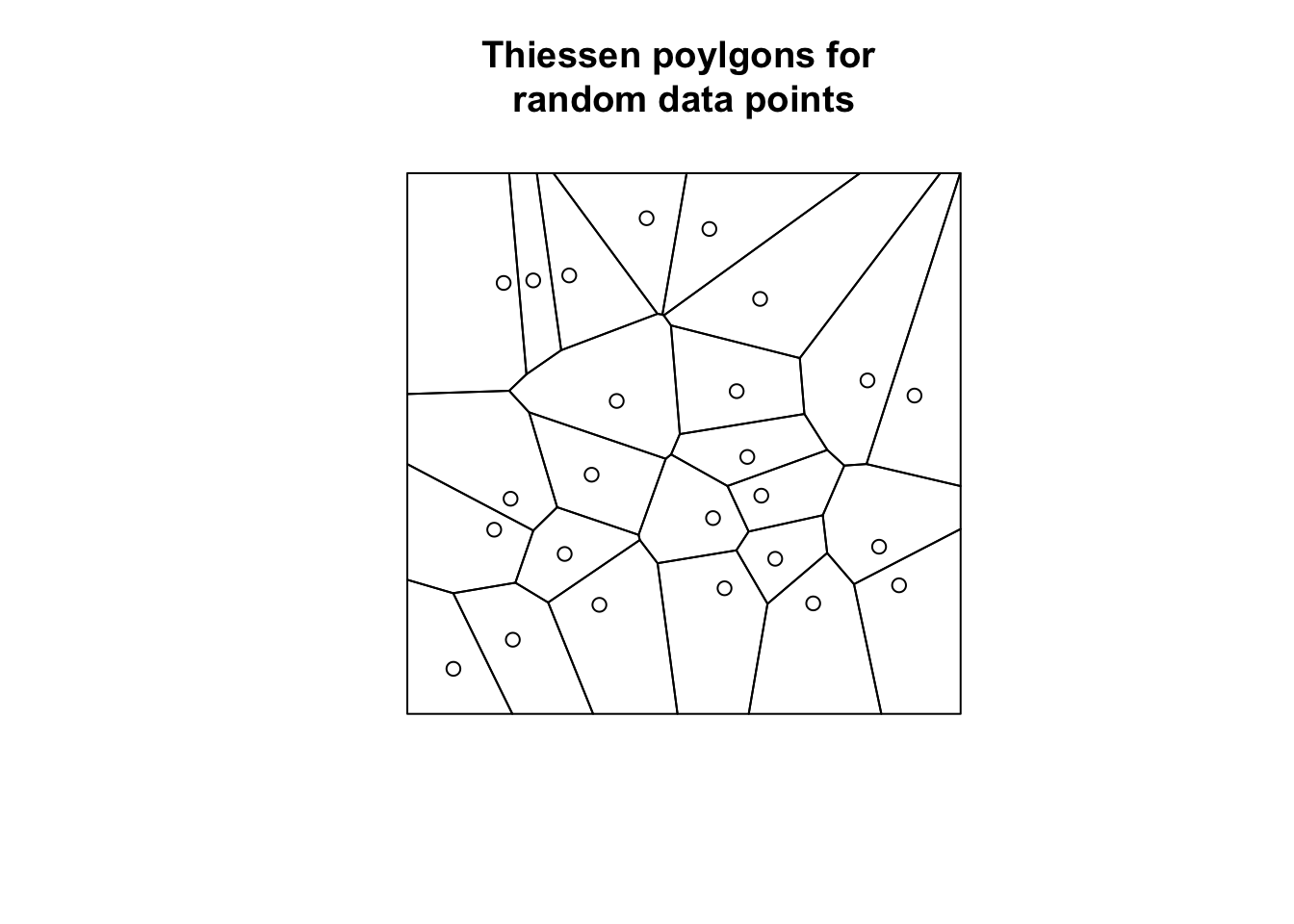The nearest neighbor interpolation (NNI) is a very simple interpolation approach. Recall that interpolation seeks for a given sample of observations $$\{z_1, z_2,..., z_n\}$$ at locations $$\{x_1, x_2,..., x_n\}$$ to estimate the value $$z$$ at some new point $$\mathbf x$$.

The NNI approach uses the value $$z_i$$ that is closest to $$\mathbf x$$. Thus, the algorithm seeks to find $$i$$ such that $$\vert \mathbf x_i - \mathbf x \vert$$ is minimized, then the estimate of $$z$$ is $$z_i$$.

It should be noted that the set of closest points to $$\mathbf x_i$$ forms a Thiessen poylgon, also known as Voronoi polygon. The technique used to compute these polygons is referred to as Delauny triangulation. Such a particular polygon corresponds to a region consisting of all points closer to $$\mathbf x_i$$ than to any other $$\mathbf x$$.

In R the dismo package, which relies on the deldir package, provides the voronoi() function to create Voronoi polygons for a set of points. The input points data set is either a SpatialPoints class object or a two column matrix with x and y coordinates.

In order to showcase the function we generate 25 random data points, build Thiessen poylgons and plot them.

library(dismo)
set.seed(3) # set seed for reproducibility

n <- 25 # number of points
x <- runif(n, -1, 1)
y <- runif(n, -1, 1)
m <- cbind(x,y)

# plotting
plot(voronoi(m), main = 'Thiessen poylgons for \nrandom data points')
points(m)It is fairly straight forward to apply this approach on a real data set. Let us compute the Voronoi polygons for the weather station data set dwd.east.sp, in particular for the mean annual temperature and the mean annual rainfall in East Germany.

We use dwd.east.sp, a spatial object of class SpatialPointsDataFrame, as input.

load(url("https://userpage.fu-berlin.de/soga/300/30100_data_sets/East_Germany.RData"))
voronoi <- voronoi(dwd.east.sp)
voronoi
## class       : SpatialPolygonsDataFrame
## features    : 66
## extent      : 140240, 528937.2, 5541058, 6107416  (xmin, xmax, ymin, ymax)
## coord. ref. : +proj=utm +zone=33 +datum=WGS84 +units=m +no_defs +ellps=WGS84 +towgs84=0,0,0
## variables   : 6
## names       : Station_id, Altitude,                 Name, Federal.State, Rainfall, Temperature
## min values  :        164,      1.2,          Angermuende,        Berlin,      305,         3.5
## max values  :       5825,   1213.0, Zinnwald-Georgenfeld,    Thueringen,     1879,        10.0

Once we computed the Voronoi polygons we may plot them by using the spplot() from the sp package.

library(gridExtra)
p1 <- spplot(voronoi, 'Temperature',
main = "Mean annual temperature [°C]") +
latticeExtra::layer(sp.polygons(east.germany.states.sp,
col = "white",
lwd = 1.5))+
latticeExtra::layer(sp.points(dwd.east.sp,
pch = 1,
col = 'black'))

p2 <- spplot(voronoi, 'Rainfall',
main = "Mean annual rainfall [mm]") +
latticeExtra::layer(sp.polygons(east.germany.states.sp,
col = "white",
lwd = 1.5))+
latticeExtra::layer(sp.points(dwd.east.sp,
pch = 1,
col = 'black'))

grid.arrange(p1, p2, ncol = 2)